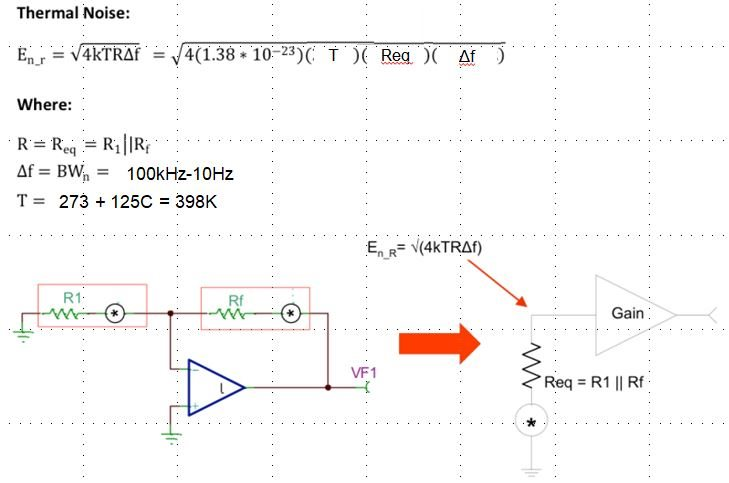If you have a related question, please click the "Ask a related question" button in the top right corner. The newly created question will be automatically linked to this question.

• Resolved

# TPS7A91: Relationship between noise performance and resistive network current (>5uA)

Part Number: TPS7A91

Hi team,

Datasheet 8.1.1 says that the current through resistive network needs >5uA for optimum noise performance.

When the resistive network current is more than 5uA, does the noise performance depend on the resistive network current? For example, the more resistive network current increases, the better the noise performance is.

Regards,

Saito

• HI Saito,

A significant source of noise in an LDO is the resistor divider network from Vout, to the FB pin, to GND. This noise is known as thermal noise and is equal to 4kTR (sometimes called 4kTR noise), where k is Boltzmann’s constant, T is temperature in Kelvin, and R is the resistance. The resistor divider is tied to the input of the LDO differential amplifier, so this noise is amplified by the closed-loop gain of the LDO. When calculating this noise source, you can simply use the parallel combination of R1 and R2 since the op amp input sees them as being virtually in parallel. Therefore, to reduce this noise source, the most important thing to remember is that smaller feedback resistors create less thermal noise. Of course, the disadvantage of using smaller resistors is that they burn more current through the feedback divider; but if noise is of prime importance, then this sacrifice must be made.

Thanks,

- Stephen

• In reply to Stephen Ziel:

Hi Stephen,

Thanks. I will try to calculate the thermal noise caused by resistor network.

How about PSRR and inherent noise in LDO?

Does these performance depend on the current through feedback resistor network?

Regards,

Saito

• In reply to Takahiro Saito:

Hi Saito,

I apologize for the delay in my response.

PSRR is mainly affected by 3 items:

1. The bandgap reference, which dominates the PSRR plot at low frequencies.
2. The internal error amplifier, which dominates the PSRR plot at midband frequencies.
3. The output capacitor, which dominates the PSRR plot at high frequencies past the gain-bandwidth of the internal error amplifier.

The bandgap reference is typically filtered by an RC low pass filter, which rolls off around 1Hz - 200Hz.
This is accomplished by an internal resistor (usually 100's of kilo-ohms) following by an external capacitor (called the NR or noise reduction capacitor).
This filter exists so that the bandgap reference noise is not gained up by the internal error amplifier, in much the same way as the external feedback resistors are (described previously).

If the feedback resistors are external, they do not typically affect intrinsic noise, except for being gained up by the internal error amplifier.
Similarly, the PSRR is not affected by them, except when a feedfoward capacitor (Cff) is placed in the output.
In that case, the feedback resistor and the feedforward capacitor form a pole that attenuates the noise and improves the PSRR.
See the following resource for more details.

http://www.ti.com/lit/an/sbva042/sbva042.pdf

A great resource that discusses the various contributions to noise in LDO's is the following link:

http://www.ti.com/lit/an/slyt201/slyt201.pdf

Thanks,

- Stephen

• In reply to Stephen Ziel:

Hi Stephen,

Thank you for the detailed explanation. I understood the current through the resistive network doesn't affect the intrinsic noise and PSRR.

I also calculated 4kRT noise based on below figure. I found that the 4kRT noise is almost equal to the intrinsic noise (4.7uVrms) when the equivarent resistor (R1//R2) is 10kohm. I will care about the value of equivalent resistor so that the 4kRT noise doen't exceed the intrinsic noise.Regards,

Saito